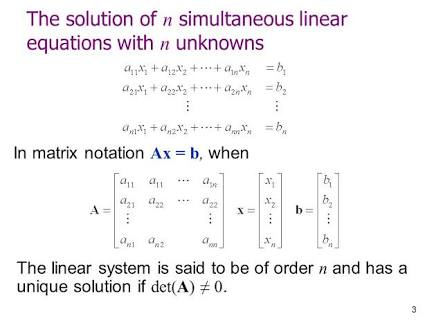Q

# Try this! - Algebra - BITSAT-8

The system of linear equation

has a unique solution if

• Option 1)

• Option 2)

• Option 3)

• Option 4)

113 Views

As learnt in

Solution of a system of equations -

satisfy the system of linear equations

- whereinFor unique solution

Option 1)

This option is correct.

Option 2)

This option is incorrect.

Option 3)

This option is incorrect.

Option 4)

This option is incorrect.

Exams
Articles
Questions